# NCERT Exemplar Solutions for Class 7 Maths Chapter 8 Rational Numbers

NCERT Exemplar Solutions for Class 7 Maths Chapter 8 Rational Numbers PDF is very useful to help students understand the question pattern and types of different problems. It is essential to understand the various kinds of problems and figure out the best solutions for them. Students can find NCERT Exemplar Solutions for Class 7 Chapter 8 and understand how to solve it.

Chapter 8 – Rational Numbers that can be expressed as the quotient or fraction p/q of two integers, a numerator p, and a non-zero denominator q. Since q may be equal to 1, every integer is a rational number. Some of the important topics covered in this Chapter are

• Positive and Negative Rational Numbers
• Rational Numbers on a Number Line
• Rational Numbers in Standard Form
• Comparison of Rational Numbers
• Operations on Rational Numbers

## Download the PDF of NCERT Exemplar Solutions for Class 7 Maths Chapter 8 Rational Numbers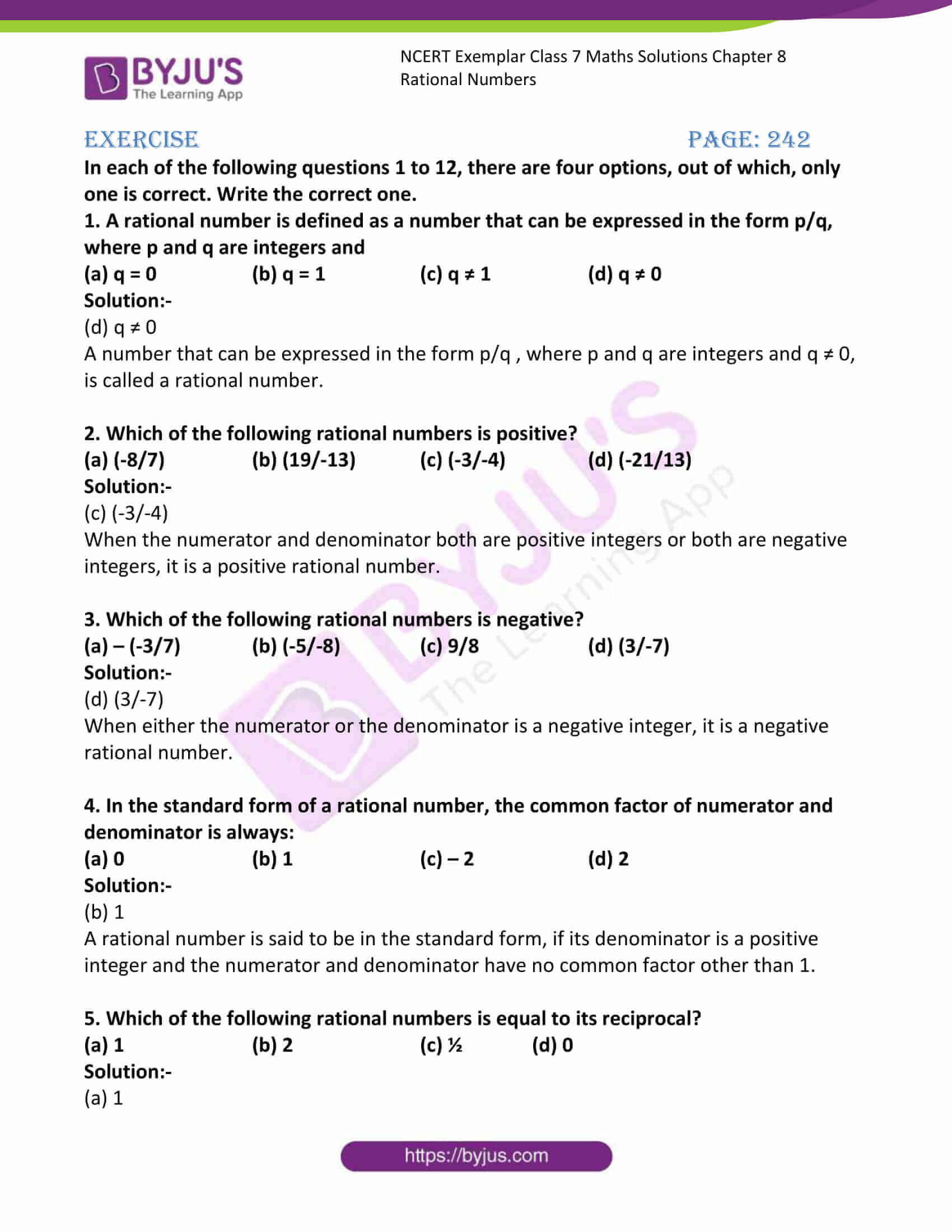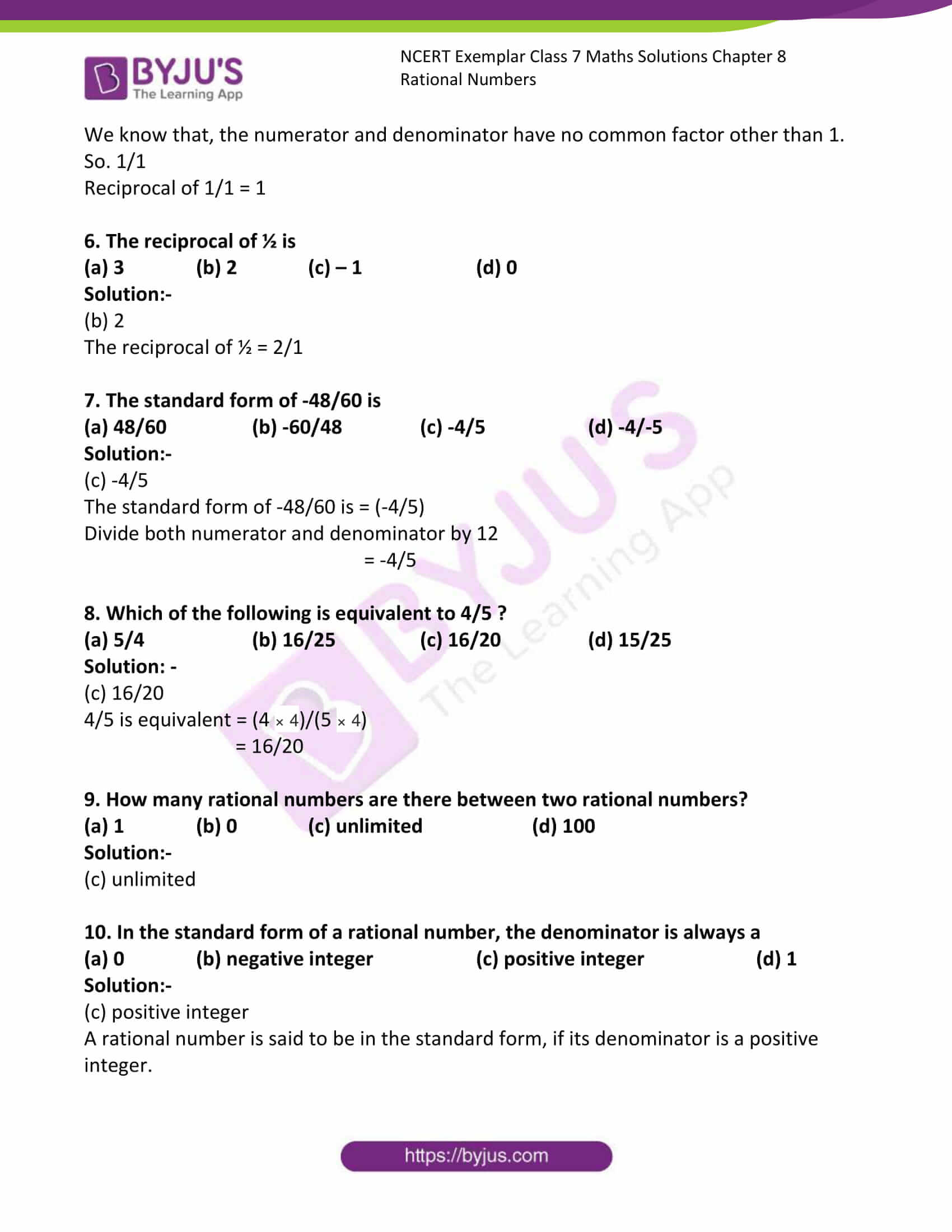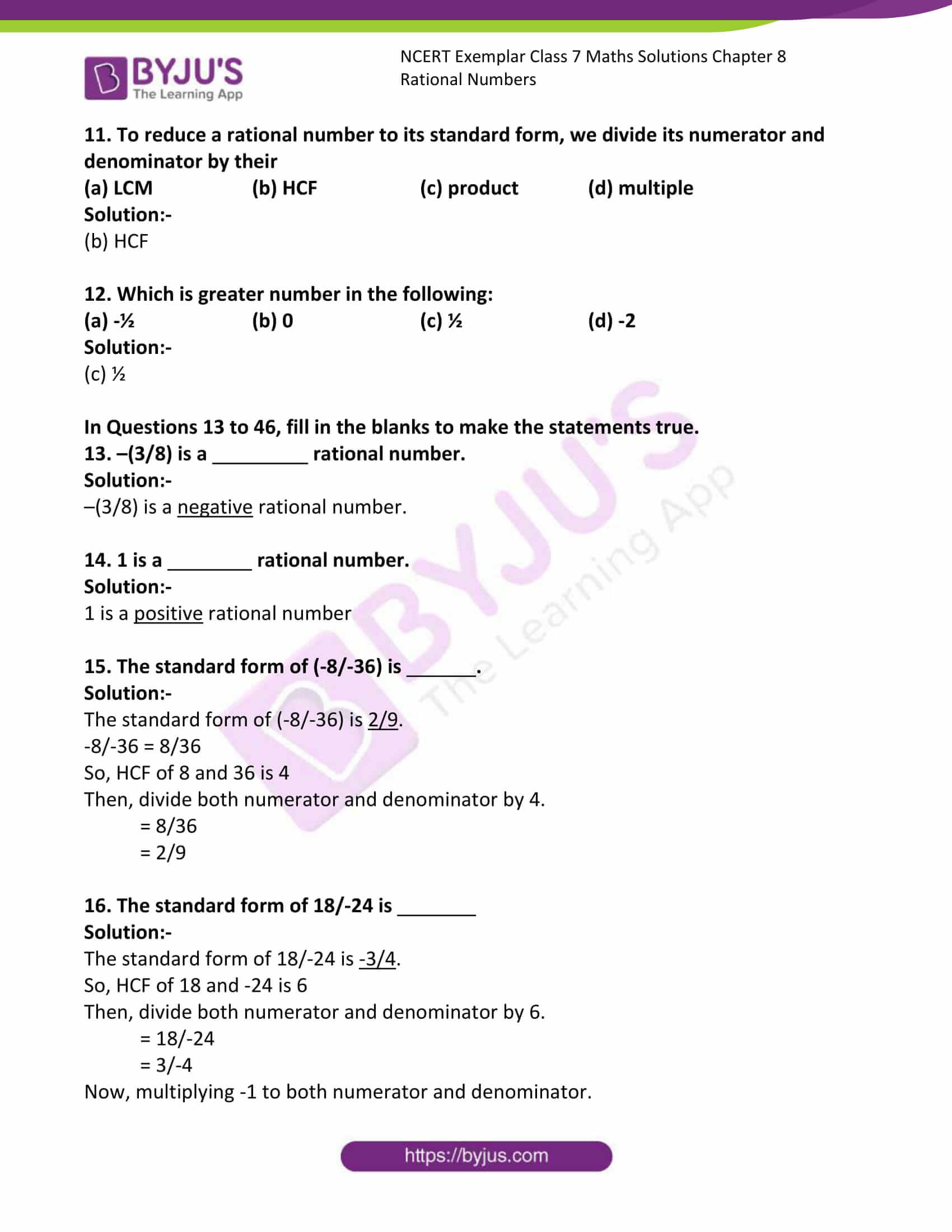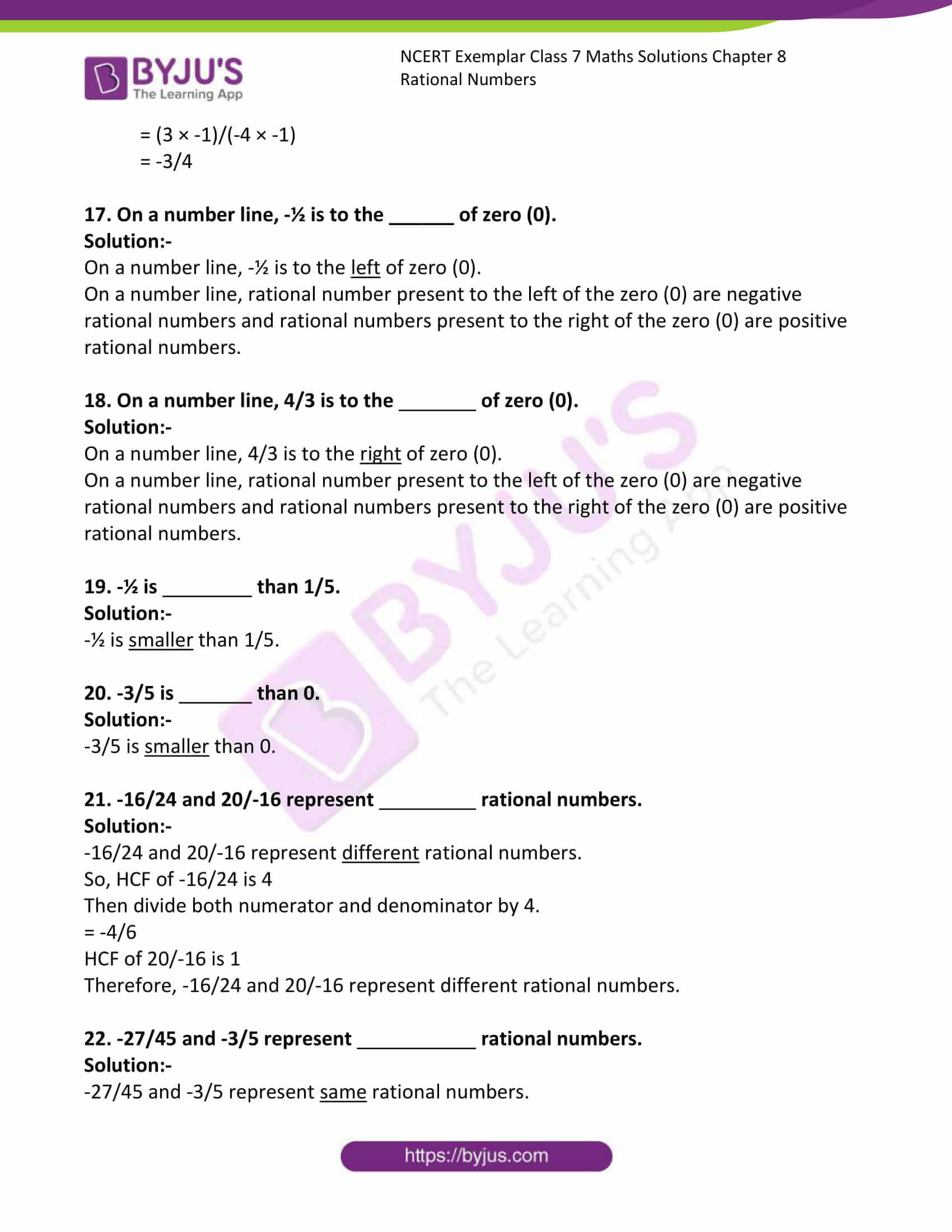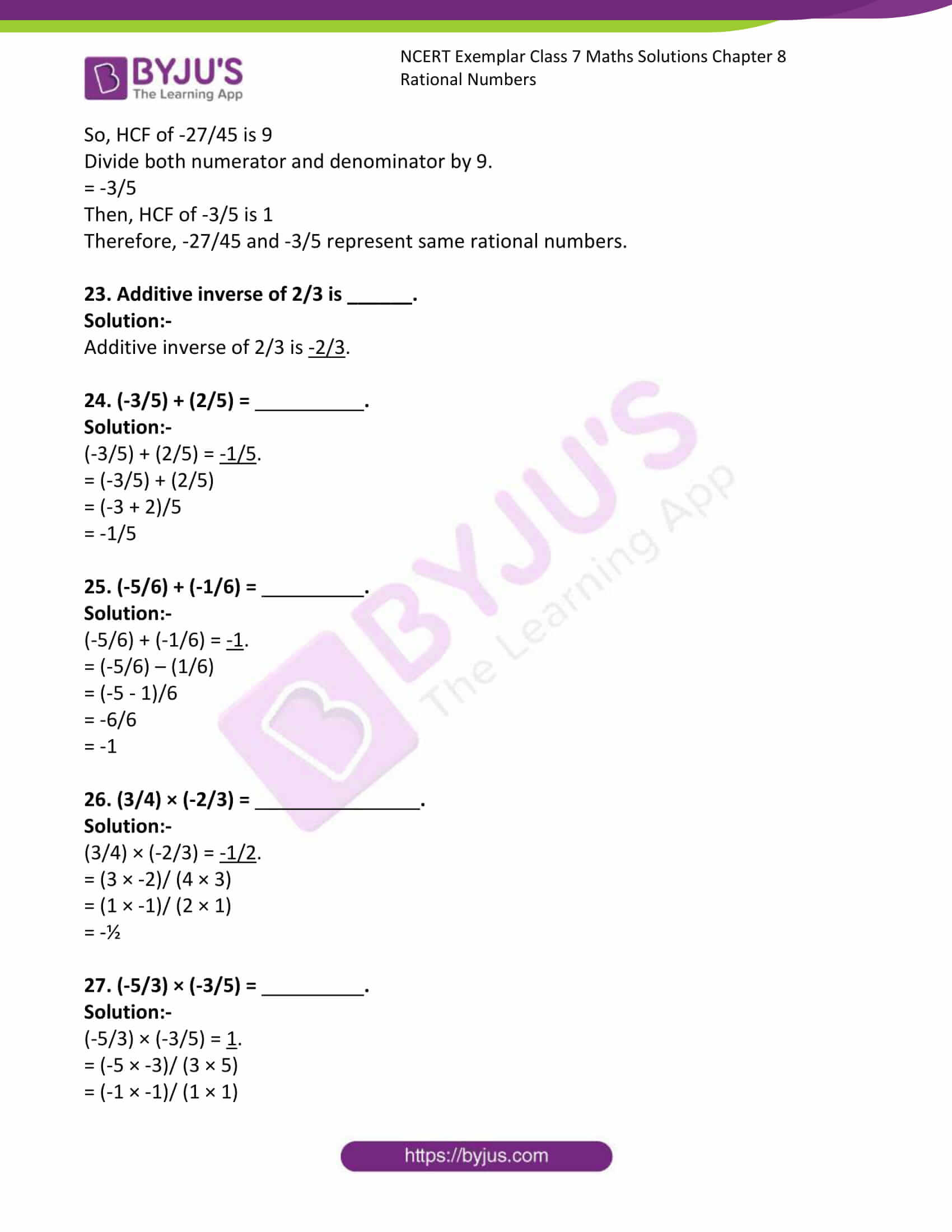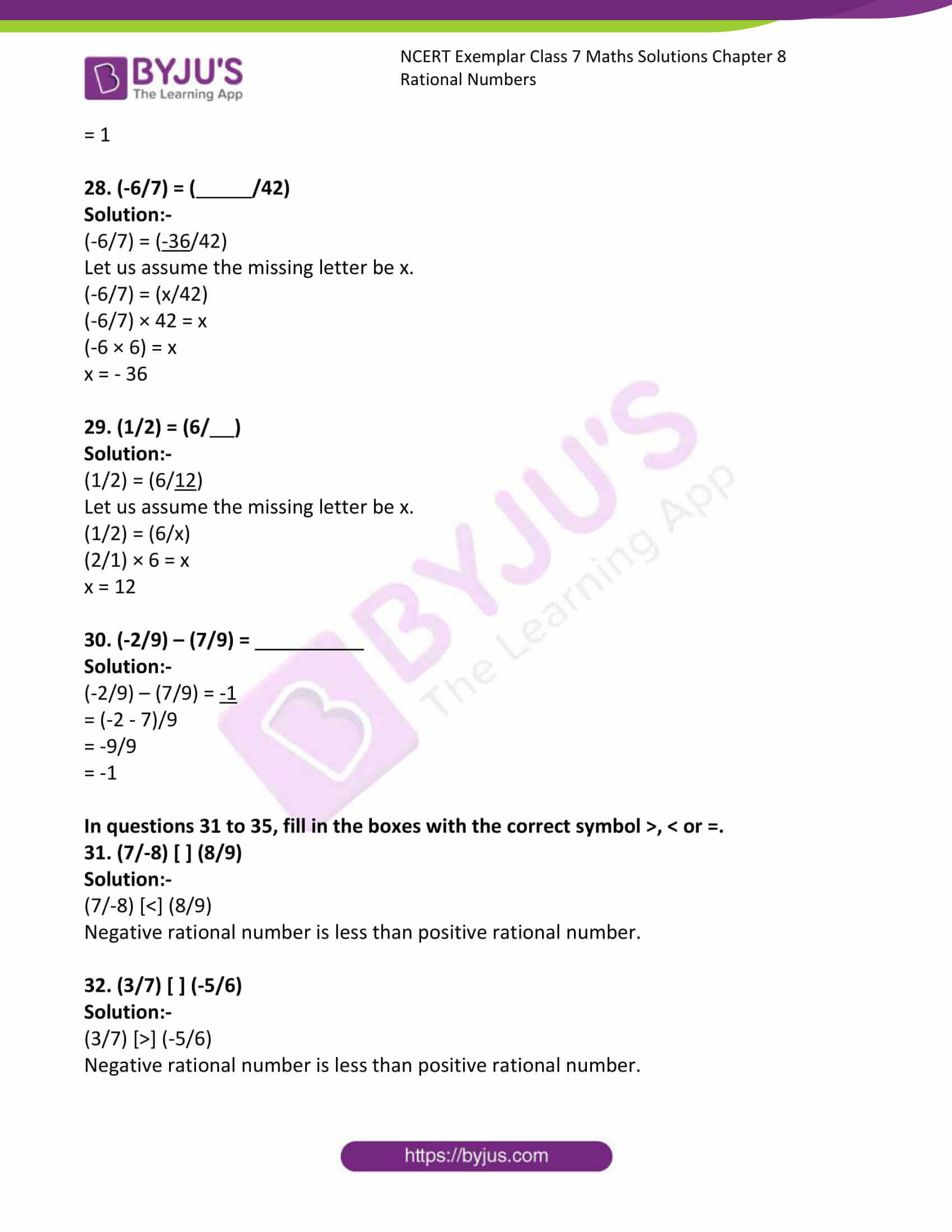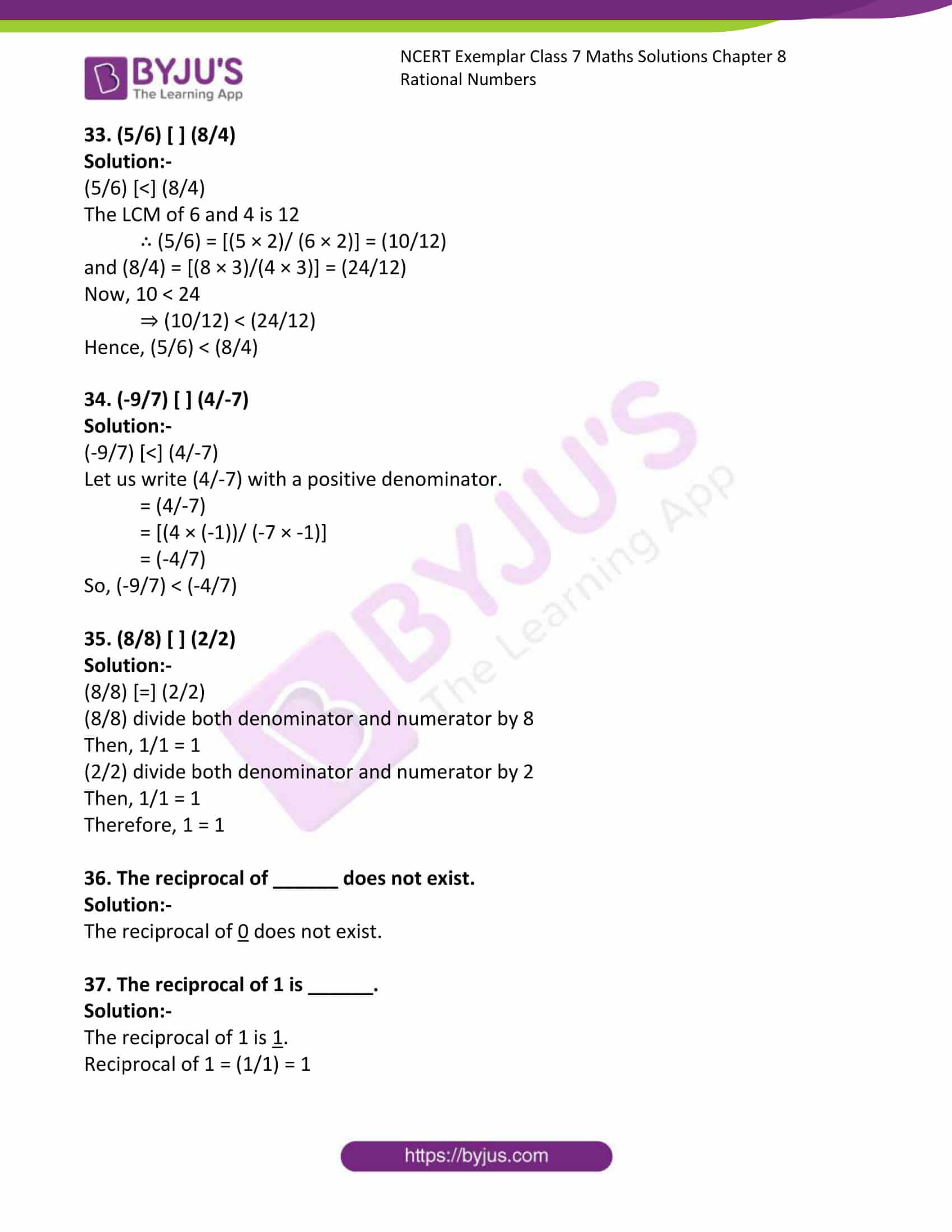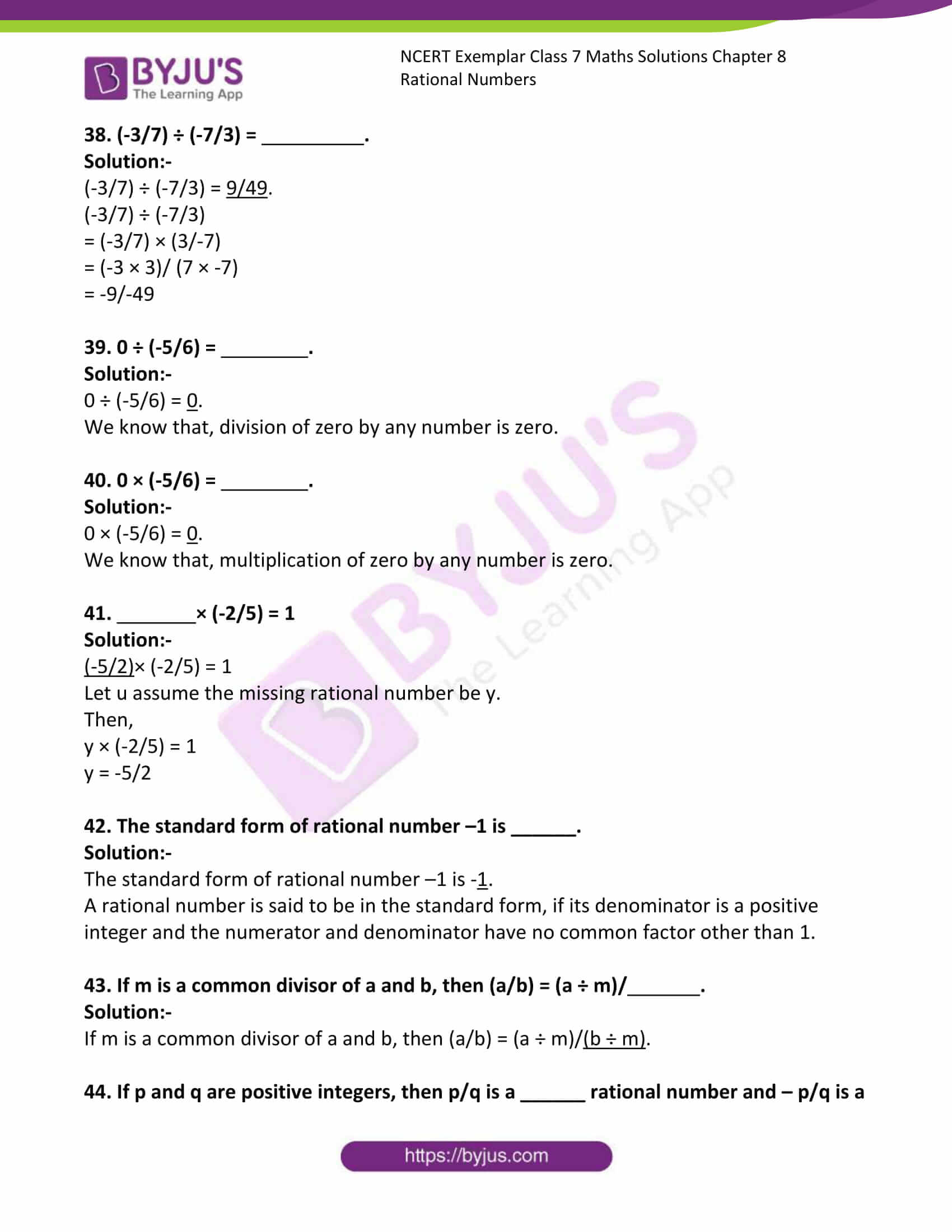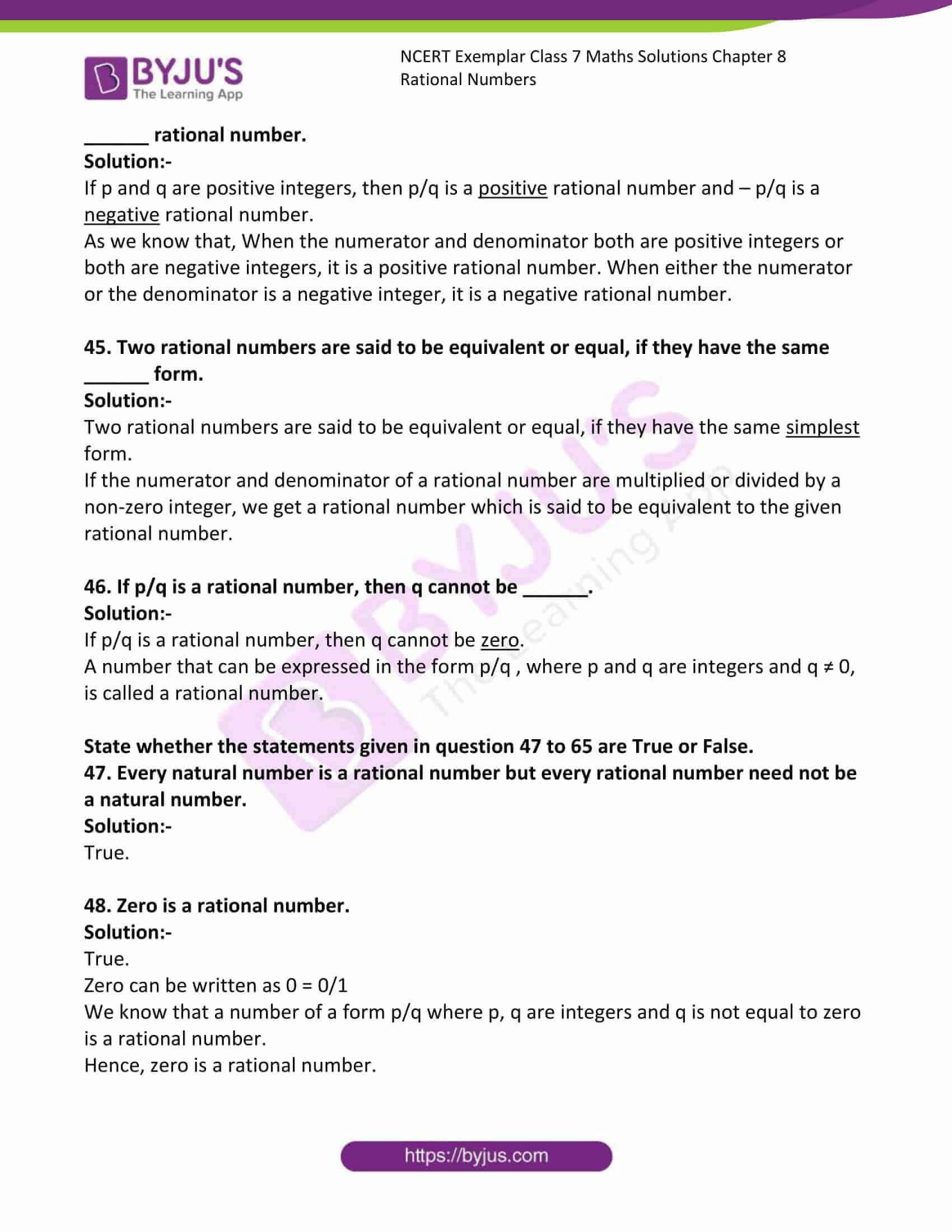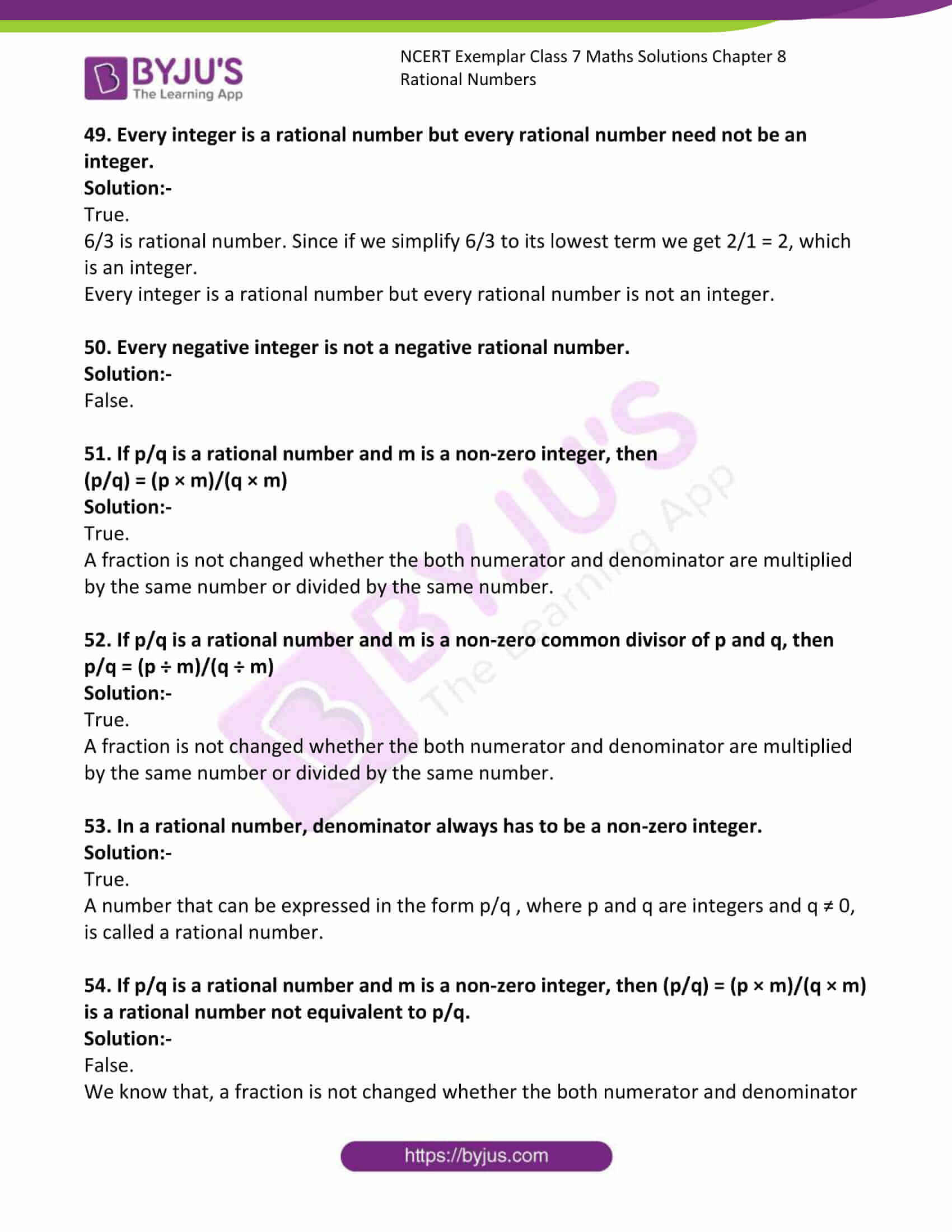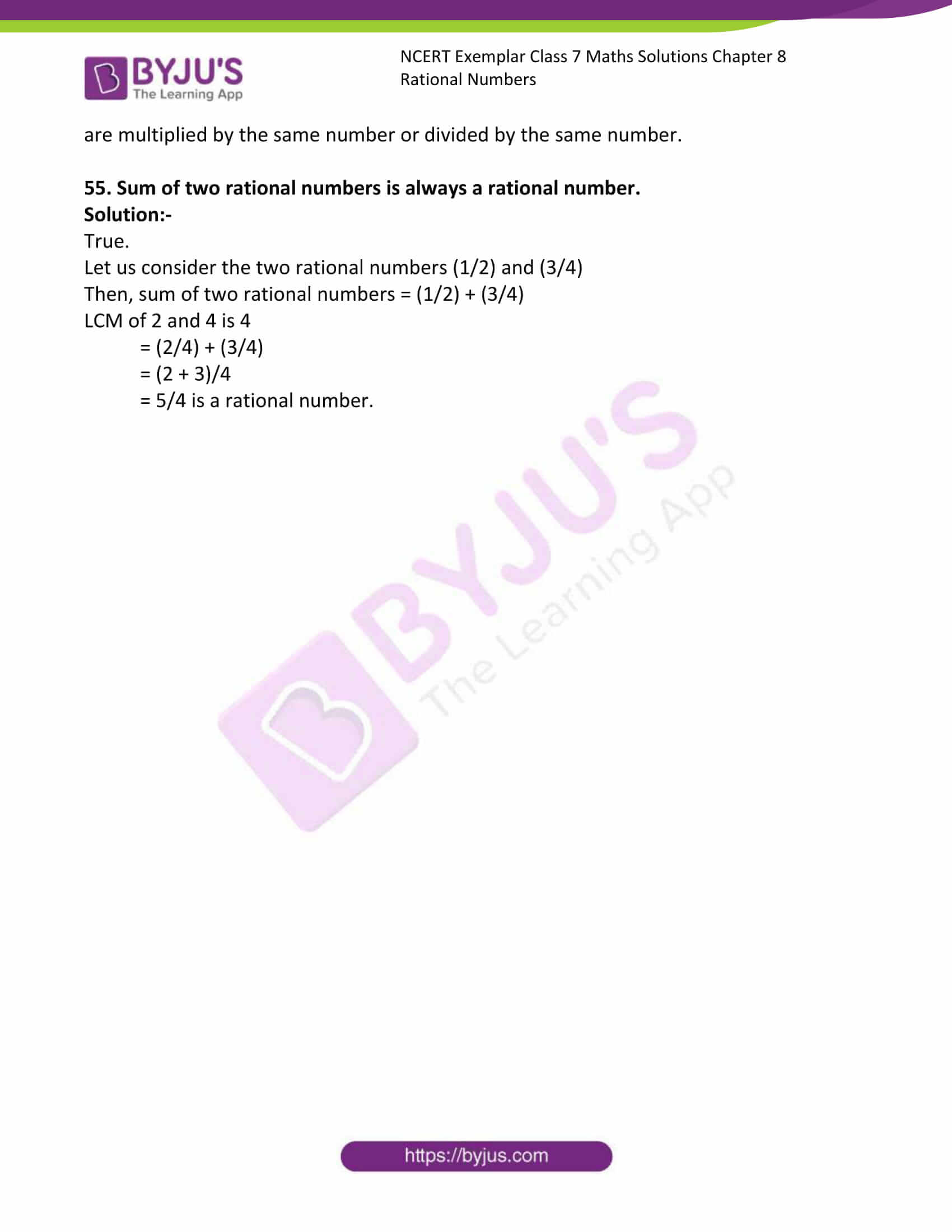### Access Answers to Maths NCERT Exemplar Solutions for Class 7 Chapter 8 Rational Numbers

Exercise Page: 242

In each of the following questions 1 to 12, there are four options, out of which, only one is correct. Write the correct one.

1. A rational number is defined as a number that can be expressed in the form p/q, where p and q are integers and

(a) q = 0 (b) q = 1 (c) q ≠ 1 (d) q ≠ 0

Solution:-

(d) q ≠ 0

A number that can be expressed in the form p/q , where p and q are integers and q ≠ 0, is called a rational number.

2. Which of the following rational numbers is positive?

(a) (-8/7) (b) (19/-13) (c) (-3/-4) (d) (-21/13)

Solution:-

(c) (-3/-4)

When the numerator and denominator both are positive integers or both are negative integers, it is a positive rational number.

3. Which of the following rational numbers is negative?

(a) – (-3/7) (b) (-5/-8) (c) 9/8 (d) (3/-7)

Solution:-

(d) (3/-7)

When either the numerator or the denominator is a negative integer, it is a negative rational number.

4. In the standard form of a rational number, the common factor of numerator and denominator is always:

(a) 0 (b) 1 (c) – 2 (d) 2

Solution:-

(b) 1

A rational number is said to be in the standard form, if its denominator is a positive integer and the numerator and denominator have no common factor other than 1.

5. Which of the following rational numbers is equal to its reciprocal?

(a) 1 (b) 2 (c) ½ (d) 0

Solution:-

(a) 1

We know that, the numerator and denominator have no common factor other than 1.

So. 1/1

Reciprocal of 1/1 = 1

6. The reciprocal of ½ is

(a) 3 (b) 2 (c) – 1 (d) 0

Solution:-

(b) 2

The reciprocal of ½ = 2/1

7. The standard form of -48/60 is

(a) 48/60 (b) -60/48 (c) -4/5 (d) -4/-5

Solution:-

(c) -4/5

The standard form of -48/60 is = (-4/5)

Divide both numerator and denominator by 12

= -4/5

8. Which of the following is equivalent to 4/5 ?

(a) 5/4 (b) 16/25 (c) 16/20 (d) 15/25

Solution: –

(c) 16/20

4/5 is equivalent = (4 × 4)/(5 × 4)

= 16/20

9. How many rational numbers are there between two rational numbers?

(a) 1 (b) 0 (c) unlimited (d) 100

Solution:-

(c) unlimited

10. In the standard form of a rational number, the denominator is always a

(a) 0 (b) negative integer (c) positive integer (d) 1

Solution:-

(c) positive integer

A rational number is said to be in the standard form, if its denominator is a positive integer.

11. To reduce a rational number to its standard form, we divide its numerator and denominator by their

(a) LCM (b) HCF (c) product (d) multiple

Solution:-

(b) HCF

12. Which is greater number in the following:

(a) -½ (b) 0 (c) ½ (d) -2

Solution:-

(c) ½

In Questions 13 to 46, fill in the blanks to make the statements true.

13. –(3/8) is a rational number.

Solution:-

–(3/8) is a negative rational number.

14. 1 is a rational number.

Solution:-

1 is a positive rational number

15. The standard form of (-8/-36) is .

Solution:-

The standard form of (-8/-36) is 2/9.

-8/-36 = 8/36

So, HCF of 8 and 36 is 4

Then, divide both numerator and denominator by 4.

= 8/36

= 2/9

16. The standard form of 18/-24 is

Solution:-

The standard form of 18/-24 is -3/4.

So, HCF of 18 and -24 is 6

Then, divide both numerator and denominator by 6.

= 18/-24

= 3/-4

Now, multiplying -1 to both numerator and denominator.

= (3 × -1)/(-4 × -1)

= -3/4

17. On a number line, -½ is to the ______ of zero (0).

Solution:-

On a number line, -½ is to the left of zero (0).

On a number line, rational number present to the left of the zero (0) are negative rational numbers and rational numbers present to the right of the zero (0) are positive rational numbers.

18. On a number line, 4/3 is to the of zero (0).

Solution:-

On a number line, 4/3 is to the right of zero (0).

On a number line, rational number present to the left of the zero (0) are negative rational numbers and rational numbers present to the right of the zero (0) are positive rational numbers.

19. -½ is than 1/5.

Solution:-

-½ is smaller than 1/5.

20. -3/5 is than 0.

Solution:-

-3/5 is smaller than 0.

21. -16/24 and 20/-16 represent rational numbers.

Solution:-

-16/24 and 20/-16 represent different rational numbers.

So, HCF of -16/24 is 4

Then divide both numerator and denominator by 4.

= -4/6

HCF of 20/-16 is 1

Therefore, -16/24 and 20/-16 represent different rational numbers.

22. -27/45 and -3/5 represent rational numbers.

Solution:-

-27/45 and -3/5 represent same rational numbers.

So, HCF of -27/45 is 9

Divide both numerator and denominator by 9.

= -3/5

Then, HCF of -3/5 is 1

Therefore, -27/45 and -3/5 represent same rational numbers.

23. Additive inverse of 2/3 is ______.

Solution:-

Additive inverse of 2/3 is -2/3.

24. (-3/5) + (2/5) = .

Solution:-

(-3/5) + (2/5) = -1/5.

= (-3/5) + (2/5)

= (-3 + 2)/5

= -1/5

25. (-5/6) + (-1/6) = .

Solution:-

(-5/6) + (-1/6) = -1.

= (-5/6) – (1/6)

= (-5 – 1)/6

= -6/6

= -1

26. (3/4) × (-2/3) = .

Solution:-

(3/4) × (-2/3) = -1/2.

= (3 × -2)/ (4 × 3)

= (1 × -1)/ (2 × 1)

= -½

27. (-5/3) × (-3/5) = .

Solution:-

(-5/3) × (-3/5) = 1.

= (-5 × -3)/ (3 × 5)

= (-1 × -1)/ (1 × 1)

= 1

28. (-6/7) = ( /42)

Solution:-

(-6/7) = (-36/42)

Let us assume the missing letter be x.

(-6/7) = (x/42)

(-6/7) × 42 = x

(-6 × 6) = x

x = – 36

29. (1/2) = (6/ )

Solution:-

(1/2) = (6/12)

Let us assume the missing letter be x.

(1/2) = (6/x)

(2/1) × 6 = x

x = 12

30. (-2/9) – (7/9) =

Solution:-

(-2/9) – (7/9) = -1

= (-2 – 7)/9

= -9/9

= -1

In questions 31 to 35, fill in the boxes with the correct symbol >, < or =.

31. (7/-8) [ ] (8/9)

Solution:-

(7/-8) [<] (8/9)

Negative rational number is less than positive rational number.

32. (3/7) [ ] (-5/6)

Solution:-

(3/7) [>] (-5/6)

Negative rational number is less than positive rational number.

33. (5/6) [ ] (8/4)

Solution:-

(5/6) [<] (8/4)

The LCM of 6 and 4 is 12

∴ (5/6) = [(5 × 2)/ (6 × 2)] = (10/12)

and (8/4) = [(8 × 3)/(4 × 3)] = (24/12)

Now, 10 < 24

⇒ (10/12) < (24/12)

Hence, (5/6) < (8/4)

34. (-9/7) [ ] (4/-7)

Solution:-

(-9/7) [<] (4/-7)

Let us write (4/-7) with a positive denominator.

= (4/-7)

= [(4 × (-1))/ (-7 × -1)]

= (-4/7)

So, (-9/7) < (-4/7)

35. (8/8) [ ] (2/2)

Solution:-

(8/8) [=] (2/2)

(8/8) divide both denominator and numerator by 8

Then, 1/1 = 1

(2/2) divide both denominator and numerator by 2

Then, 1/1 = 1

Therefore, 1 = 1

36. The reciprocal of ______ does not exist.

Solution:-

The reciprocal of 0 does not exist.

37. The reciprocal of 1 is ______.

Solution:-

The reciprocal of 1 is 1.

Reciprocal of 1 = (1/1) = 1

38. (-3/7) ÷ (-7/3) = .

Solution:-

(-3/7) ÷ (-7/3) = 9/49.

(-3/7) ÷ (-7/3)

= (-3/7) × (3/-7)

= (-3 × 3)/ (7 × -7)

= -9/-49

39. 0 ÷ (-5/6) = .

Solution:-

0 ÷ (-5/6) = 0.

We know that, division of zero by any number is zero.

40. 0 × (-5/6) = .

Solution:-

0 × (-5/6) = 0.

We know that, multiplication of zero by any number is zero.

41. × (-2/5) = 1

Solution:-

(-5/2)× (-2/5) = 1

Let u assume the missing rational number be y.

Then,

y × (-2/5) = 1

y = -5/2

42. The standard form of rational number –1 is ______.

Solution:-

The standard form of rational number –1 is -1.

A rational number is said to be in the standard form, if its denominator is a positive integer and the numerator and denominator have no common factor other than 1.

43. If m is a common divisor of a and b, then (a/b) = (a ÷ m)/ .

Solution:-

If m is a common divisor of a and b, then (a/b) = (a ÷ m)/(b ÷ m).

44. If p and q are positive integers, then p/q is a ______ rational number and – p/q is a ______ rational number.

Solution:-

If p and q are positive integers, then p/q is a positive rational number and – p/q is a negative rational number.

As we know that, When the numerator and denominator both are positive integers or both are negative integers, it is a positive rational number. When either the numerator or the denominator is a negative integer, it is a negative rational number.

45. Two rational numbers are said to be equivalent or equal, if they have the same ______ form.

Solution:-

Two rational numbers are said to be equivalent or equal, if they have the same simplest form.

If the numerator and denominator of a rational number are multiplied or divided by a non-zero integer, we get a rational number which is said to be equivalent to the given rational number.

46. If p/q is a rational number, then q cannot be ______.

Solution:-

If p/q is a rational number, then q cannot be zero.

A number that can be expressed in the form p/q , where p and q are integers and q ≠ 0, is called a rational number.

State whether the statements given in question 47 to 65 are True or False.

47. Every natural number is a rational number but every rational number need not be a natural number.

Solution:-

True.

48. Zero is a rational number.

Solution:-

True.

Zero can be written as 0 = 0/1

We know that a number of a form p/q where p, q are integers and q is not equal to zero is a rational number.

Hence, zero is a rational number.

49. Every integer is a rational number but every rational number need not be an integer.

Solution:-

True.

6/3 is rational number. Since if we simplify 6/3 to its lowest term we get 2/1 = 2, which is an integer.

Every integer is a rational number but every rational number is not an integer.

50. Every negative integer is not a negative rational number.

Solution:-

False.

51. If p/q is a rational number and m is a non-zero integer, then

(p/q) = (p × m)/(q × m)

Solution:-

True.

A fraction is not changed whether the both numerator and denominator are multiplied by the same number or divided by the same number.

52. If p/q is a rational number and m is a non-zero common divisor of p and q, then

p/q = (p ÷ m)/(q ÷ m)

Solution:-

True.

A fraction is not changed whether the both numerator and denominator are multiplied by the same number or divided by the same number.

53. In a rational number, denominator always has to be a non-zero integer.

Solution:-

True.

A number that can be expressed in the form p/q , where p and q are integers and q ≠ 0, is called a rational number.

54. If p/q is a rational number and m is a non-zero integer, then (p/q) = (p × m)/(q × m) is a rational number not equivalent to p/q.

Solution:-

False.

We know that, a fraction is not changed whether the both numerator and denominator are multiplied by the same number or divided by the same number.

55. Sum of two rational numbers is always a rational number.

Solution:-

True.

Let us consider the two rational numbers (1/2) and (3/4)

Then, sum of two rational numbers = (1/2) + (3/4)

LCM of 2 and 4 is 4

= (2/4) + (3/4)

= (2 + 3)/4

= 5/4 is a rational number.